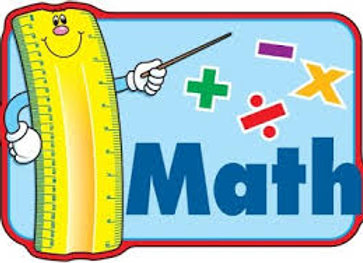# Algebra 1 A/B

`The Algebra 1 A/B Course is designed to explore, analyze, and model real-world phenomena through a mathematical lens. Exploration of linear, exponential, and quadratic functions forms the foundation of the course. Students develop conceptual understanding and fluency in solving equations, inequalities, and systems by explaining and validating their reasoning with increased precision. Students deepen their understanding of functions and their ability to represent, interpret, and communicate about them. Key characteristics and representations of functions—graphic, numeric, symbolic, and verbal—are analyzed and compared. Students use these representations to model relationships and constraints, but also reason with them abstractly. One- and two-variable data sets are interpreted using mathematical models. Gathering and displaying data, measuring data distribution, and interpreting statistical results encourages students to collaborate, communicate, an explore new tools and routines. They then take those insights to a unit on two-variable statistics, where they extend their prior knowledge of scatter plots and lines of best fit. Throughout the units of study, classroom activities provide students with opportunities to engage in aspects of mathematical modeling. Modeling prompts are used so that students experience and engage in the full modeling cycle.`